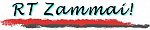Sitemap | Coverstory | Photos | Railways z. | Bridges z. | Seeds
\$B8=:_\$N%Z!<%8(B :
 \$B%H%C%W(B >> \$B%P!<%_%s%,%`JX\$j(B >> 2001\$BG/(B >> 4-6\$B7n(B >> \$B!V1~Jg!&A*9M!W(B
You are now in :
 Top >> From Birmingham >> Yr. 2001 >> 2nd Q. >> "Application & selection"

2001. 8. 27\$B5-(B
written 27 August 2001

# \$B%P!<%_%s%,%`JX\$j!V1~Jg!&A*9M!W(B From Birmingham --- Application and selection

 After spending three years with the University of Tokyo and another three years with Tokyo Electric Power Company, Inc., I had been looking for the next position to work with. Thanks to Dr Goodman who introduced me the vacancy for the TICO (Transport InterChange Optimisation) project in his research group with the University of Birmingham, I applied for the post and was successful in taking it. One of the most interesting things around this application should be the interview done over the international telephone line. Before the interview I sent a Microsoft PowerPoint presentation data by e-mail; The interview was done using the telephone, looking at the same presentation data on both ends of the line.

\$BKM\$O!"(B \$BEl5~Bg3X\$HEl5~EENO\$G\$=\$l\$>\$l(B3\$BG/\$:\$D!"(B \$B9g7W(B6\$BG/4V\$K\$o\$?\$C\$F!"(B \$B9qFb\$G8&5f6HL3\$K=>;v\$7\$F\$-\$?!#(B \$B\$I\$A\$i\$N7@Ls\$bM-4|8B7@Ls\$G\$"\$C\$?\$+\$i!"(B 2001\$BG/(B3\$B7n\$K=*N;\$9\$kEl5~EENO\$H\$N7@Ls\$N(B \$B!VuBV\$@\$C\$?\$o\$1\$G\$"\$k!#(B \$B\$7\$+\$7!"(B \$BF|K\\$G\$b\$\$\$h\$\$\$hBg5,LO%j%9%H%i\$,K\3J2=\$9\$k\$h\$&\$K\$J\$C\$?\$+\$i!"(B \$B\$3\$&\$7\$?\$3\$H\$rC&K!9T0Y\$HHcH=\$G\$-\$?\$N\$b2{\$+\$7\$\$@N!"(B \$B\$H\$\$\$&;~Be\$KF~\$k\$N\$@\$m\$&!#(B

\$B\$H\$b\$"\$l!"(B \$B\$=\$N8e\$K\$D\$\$\$F!"(B \$B\$+\$J\$jA0\$+\$i(B \$B!V\$=\$&\$\$\$&\$3\$H\$,\$"\$C\$?\$i\$h\$m\$7\$/\$M!W(B \$B\$H\$*4j\$\$\$r\$7\$F\$\$\$?!"(B \$B1Q9q(B The University of Birmingham \$B!J%P!<%_%s%,%`Bg3X!K(B \$B\$N(B Dr. Colin J. Goodman \$B!J@lLg\$OEE5\$E4F;!K(B \$B\$KLd\$\$9g\$o\$;\$r\$7\$?\$H\$3\$m!"(B 3\$B7n%9%?!<%H\$N(B TICO (Transport Interchange Optimisation) \$B%W%m%8%'%/%H\$G8&5f0w\$rJg=8\$9\$k!"(B \$B\$H\$N>pJs\$r\$\$\$?\$@\$-!"(B \$B1~Jg\$7\$?\$i:NMQ\$5\$l\$?!"(B \$B\$H\$\$\$&\$N\$,!"(B \$B:#2s\$N:NMQ\$N\$\$\$-\$5\$D\$NHs>o\$K4JC1\$JMWLs\$G\$"\$k!#(B

Dr Goodman \$B\$O!"(B \$BKM\$,ElBg\$K6PL3\$7\$F\$\$\$?\$H\$-!"(B \$BElBg\$,>7f[\$7!"(B \$BKM\$,\$*@\$OC\$r\$7\$?5R0w65uBV\$GA*9M\$r;O\$a\$k\$3\$H\$O!"(B \$B\$"\$N%"%a%j%+\$b4^\$a!"(B \$B\$I\$3\$N9q\$G\$b\$^\$:\$J\$\$\$i\$7\$\$!#(B \$B\$3\$l\$O!"(B \$B\$?\$H\$(\$P%9%]!<%DA*/\$J\$/!"(B \$B4uK>l9g\$N:NMQ\$H\$O<+\$:\$H0[\$J\$k\$o\$1\$G\$"\$k!#(B

\$B\$b\$A\$m\$s!"(B \$B8x@5\$J \$B\$A\$J\$_\$K!"(B \$B\$=\$N8xJg9-9p\$,\$^\$:=P\$k>l=j\$O!"(B \$B%\$%s%?!<%M%C%H\$N%&%'%V%Z!<%8\$G\$"\$C\$?!#(B \$B1~Jg=qN`\$N=q<0\$b(B Microsoft Word \$BJ8=q%U%!%\$%k\$N7A<0\$G8x3+\$5\$l\$F\$*\$j!"(B \$B%@%&%s%m!<%I\$7\$F\$=\$l\$r%F%s%W%l!<%H\$K\$7\$F:n@.\$7!"(B \$BJVAw\$9\$l\$P\$h\$\$!#(B PDF \$B\$G\$b8x3+\$5\$l\$F\$\$\$k\$+\$i!"(B \$B\$3\$l\$r%@%&%s%m!<%I!&0u:~\$7!"(B \$B \$B\$=\$N8e!"(B \$B=qN`?3::\$,9T\$o\$l\$?8eLL@\(B (interview) \$B\$,9T\$o\$l\$?\$,!"(B \$B\$3\$l\$O(B Dr Goodman \$B\$N\$4Ds0F\$G9q:]EEOC%\$%s%?%S%e!<\$H\$\$\$&7A<0\$G9T\$o\$l\$?!#(B \$BM=\$a(B Microsoft PowerPoint \$B\$N%W%l%<%s%F!<%7%g%s%G!<%?\$rF|K\\$+\$iEE;R%a!<%k\$GAw?.\$7\$F\$*\$/!#(B \$B%\$%s%?%S%e! \$B\$A\$J\$_\$K!"(B \$B1Q9qB&\$+\$i\$NDs0F\$NCf\$K\$O(B "video conferencing facility" \$B\$O\$J\$\$\$+!"(B \$B\$H\$\$\$&\$N\$b\$"\$C\$?!#(B \$BMW\$9\$k\$K%\$%s%?!<%M%C%H7PM3\$GF02h\$r\$d\$j\$H\$j\$7\$J\$,\$iLL@\\$9\$k!"(B \$B\$H\$\$\$&\$b\$N\$G\$"\$k!#(B \$B8_49@-LdBj\$,5\$\$K\$J\$C\$FF'\$_@Z\$l\$J\$+\$C\$?\$,!"(B \$B\$3\$l\$,\$G\$-\$?\$J\$i\$b\$C\$HLLGr\$\$BN83\$,\$G\$-\$?\$K0c\$\$\$J\$\$!#(B

\$B9bLZ(B \$BN<(B / TAKAGI, Ryo webmaster@takagi-ryo.ac